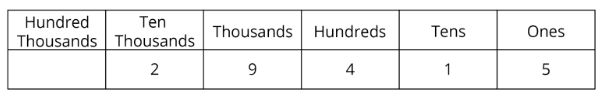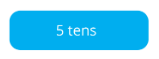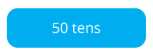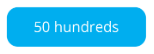Study P4 Mathematics Maths - Whole Numbers - Geniebook# Whole Numbers

2. Identifying the place values of numbers
3. Identifying the values of numbers

## 1. Reading And Writing Numbers

In Primary 4, we will learn about numbers up to 100 000.

Just like in Primary 3, when writing numbers greater  than 1000 in words, we have to separate the hundreds from the thousands with a comma.

Question 1:

Write the following in words.Twelve thousand, five hundred and sixty

Question 2:One hundred thousand

Question 3:

Write the following in words.Fifteen thousand, two hundred and twenty-five

Question 4:

Write the following in words.Twenty-one thousand and twenty–one

Question 5:

Write the following in words.Forty–two thousand, five hundred and ninety-eight

Question 6:

Write the following in words.Forty-three thousand and eleven

Question 7:

Write the following in numerals.15 310

Question 8:

Write the following in numerals.13 012

Question 9:

Write the following in numerals.70 817

Question 10:

Write the following in numerals.63 847

## 2. Identifying The Place Values Of Numbers

Let’s place the digits in 29 415 in their respective place values in the table below.The digit 5 is in the ones place.

The digit 1 is in the tens place.

The digit 4 is in the hundreds place.

The digit 9 is in the thousands place.

The digit 2 is in the ten thousands place.

Note: Remember to write the ‘s’ at the end of the place values!

Question 1:

In the following number, the digit 9 is in the __________ place.Solution:

Identify the place value of each of the digits in the following number.

The digit 0 is in the ones place.

The digit 2 is in the tens place.

The digit 7 is in the hundreds place.

The digit 9 is in the thousands place.

The digit 6 is in the ten thousands place.

thousands

Question 2:

In the following number, the digit 7 is in the __________ place.ten thousands

Question 3:

In the following number, the digit 0 is in the __________ place.hundreds

## 3. Identifying The Values Of Numbers

Let’s place the digits in 29 415 in their respective places in the table below.Depending on where the digits are in the table, they each represent a different value.

The digit 5 is in the ones place, it has a value of 5.

The digit 1 is in the tens place, it has a value of 10.

The digit 4 is in the hundreds place, it has a value of 400.

The digit 9 is in the thousands place, it has a value of 9000.

The digit 2 is in the ten thousands place, it has a value of 20 000.

Question 1:

In the following number, the value of the digit 4 is __________.Solution:

Since the digit 4 is in the ten thousands place, it has a value of 40 000.

40 000

Question 2:

In the following number, the value of the digit 6 is __________.Solution:

Since the digit 6 is in the hundreds place, it has a value of 600.

600

Question 3:

In the following number, the value of the digit 8 is __________.Solution:

Since the digit 8 is in the thousands place, it has a value of 8000.

8000

Question 4:

Which of the following has the same value as the number shown?1. $$4000 + 600 + 500 + 80$$
2. $$4600 + 500 + 80$$
3. $$40\; 000 + 6000 + 500 + 80$$
4. $$40 \;000 + 6000 + 5000 + 80$$

Solution:

\begin{align}​​ 4000 + 600 + 500 + 80 &= 5180 \\[2ex] 4600 + 500 + 80 &= 5180\\[2ex] 40 \;000 + 6000 + 500 + 80 &= 46 \;580\\[2ex] 40 \;000 + 6000 + 5000 + 80 &= 51 \;080 \end{align}

(3) 46 580

Question 5:

In which of the following numbers is the value of the digit ‘7’ the greatest?Solution:

The value of the digit ‘7’ in 21 570 is 70.

The value of the digit ‘7’ in 702 is 700.

The value of the digit ‘7’ in 1079 is 70.

The value of the digit ‘7’ in 907 is 7.

(2) 702

Question 6:

What is the sum of the values of the digit ‘2’ in the following numbers?1. 220
2. 222
3. 2220
4. 2222

Solution:

The value of the digit ‘2’ in 11 250 is 200.

The value of the digit ‘2’ in 2680 is 2000.

The value of the digit ‘2’ in 928 is 20.
Sum of the values of the digit ‘2’ of the above numbers \begin{align}​​\\[2ex] &= 200 + 2000 + 20\\[2ex] &=2220 \end{align}

(3) 2220

Question 7:

What is the value of the following?

 A)B)C)Solution:

A) 5 tens stands for 50.

50

B) 50 tens stands for 500.

500

C) 50 hundreds stands for 5000.

5000

Question 8:

What is the value of the following?1. 493
2. 1213
3. 4813
4. 4830

Solution:

4 thousands $$= 4000$$

8 hundreds  $$= 800$$

13 ones  $$= 13$$
4 thousands 8 hundreds 13 ones \begin{align}​​\\ &= 4000 + 800 + 13\\ &=4813 \end{align}

(3) 4813

Continue Learning
Multiplication Whole Numbers
Multiplication And Division Decimals
Model Drawing Strategy Division
Fractions Factors And Multiples
Area And Perimeter 1 Line Graphs
TimePrimaryPrimary 1Primary 2Primary 3Primary 4EnglishMaths
Multiplication
Whole Numbers
Line Graphs
Time
Multiplication And Division
Decimals
Model Drawing Strategy
Division
Fractions
Factors And Multiples
Area And Perimeter 1SciencePrimary 5Primary 6SecondarySecondary 1Secondary 2Secondary 3Secondary 4